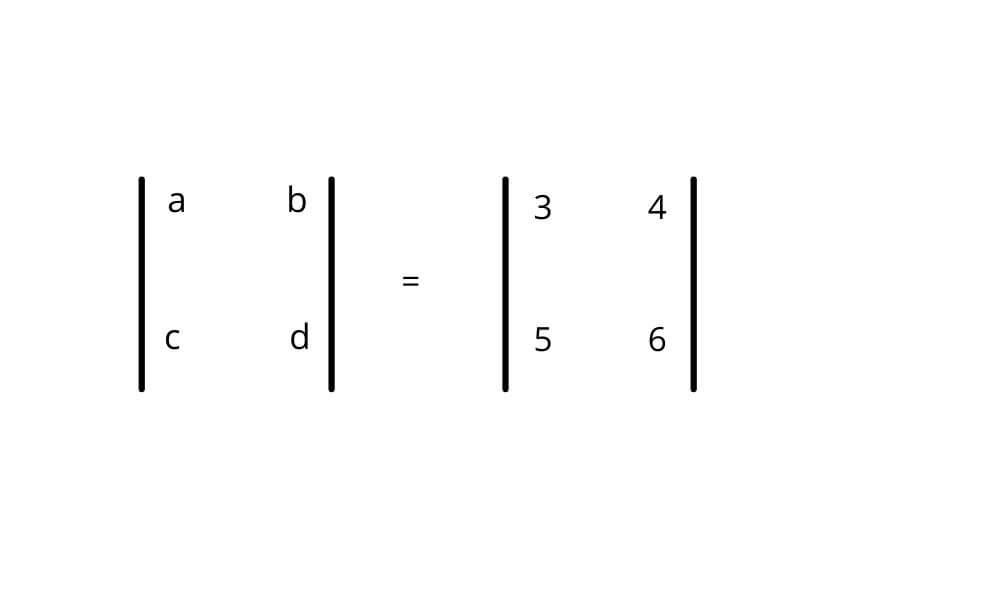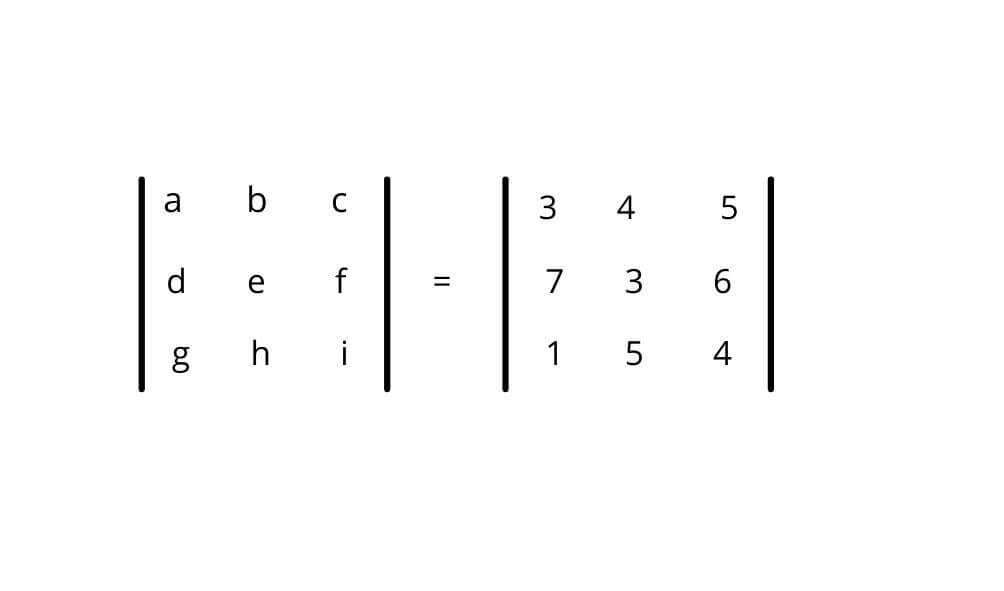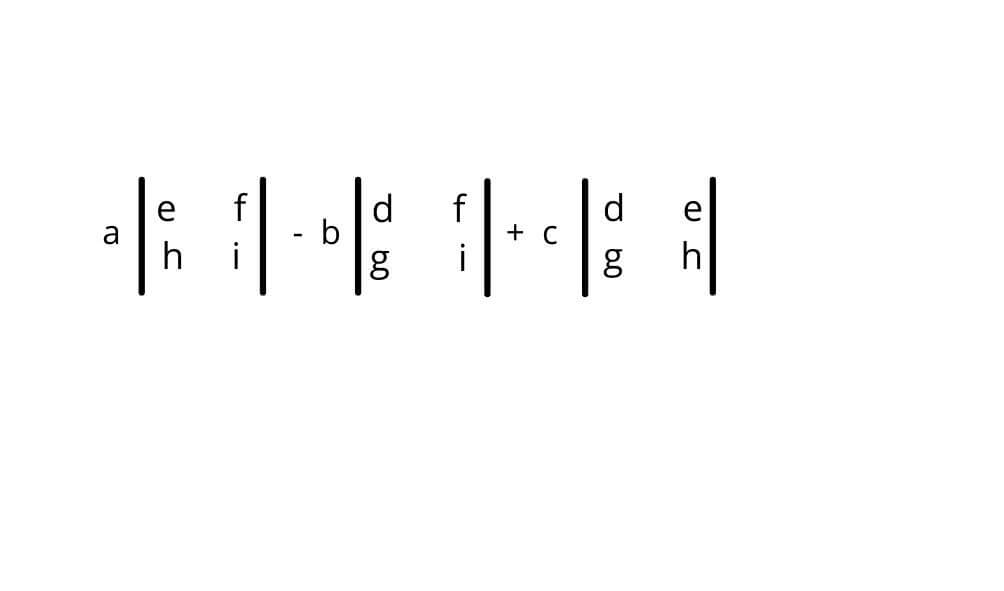# Determinant Calculator

To compute the determinant, enter the values of a matrix and select its order in the Determinant of a matrix calculator.## Matrix Determinant Calculator

This determinant calculator can find the determinant of the matrix of order 2*2, 3*3, 4*4, and 5*5. The modern design of this calculator allows entering values easily without error.

## Definition - Determinant of the matrix

A determinant is a function of the scalar matrix which gives certain information about the matrix. This information is related to the inverse of a matrix, area of triangle e.t.c.## How to find the determinant of a matrix?

The method of determinant differs for each order. Below are the methods for computing determinants of the matrix of order 22 and 33.

## Determinant of matrix 2 by 2:Determinant = (a)(d) - (b)(c)

= (3)(6) - (4)(5)

= -2

## Determinant of matrix 3 by 3:The formula of 3 by 3 matrix is:

=a {(e * i)-(f*h)}-b {(d*i)-(f*g)}+c{(d*h)-(e*g)}

Put the values in the formula:= 3 {( 3*4 ) - ( 6*5 )} - 4 {(7*4 ) - ( 6*1 )} + 5 {( 7*5 ) - ( 3*1 )}

= 3 (-18) - 4 (22) + 5 (32)

= - 54 - 88 + 160

= -142 + 160

= 18